# Detect Image Anomalies Using Explainable One-Class Classification Neural Network

This example shows how to train an anomaly detector for visual inspection of pill images.

In one-class anomaly detection approaches, training is semisupervised, meaning that the network trains on data consisting only of normal images without anomalies . Despite training on samples only of normal scenes, the model learns how to distinguish between normal and anomalous scenes. One-class learning offers many advantages for anomaly detection problems:

• Representations of anomalies can be scarce.

• Anomalies can represent expensive or catastrophic outcomes.

• There can be many kinds of anomalies and the kinds of anomalies can change over the lifetime of the model. Describing what "good" looks like is often more feasible than providing data that represents all possible anomalies in real world settings.

A crucial goal of anomaly detection is for a human observer to be able to understand why a trained network classifies images as anomalies. Explainable classification supplements the class prediction with information that justifies how the neural network reached its classification decision.

This example explores how one-class deep learning can be used to create effective anomaly detectors. The example also implements explainable classification using a network that returns a heatmap with the probability that each pixel is anomalous. The classifier labels images as normal or anomalous based on the mean value of the anomaly score heatmap.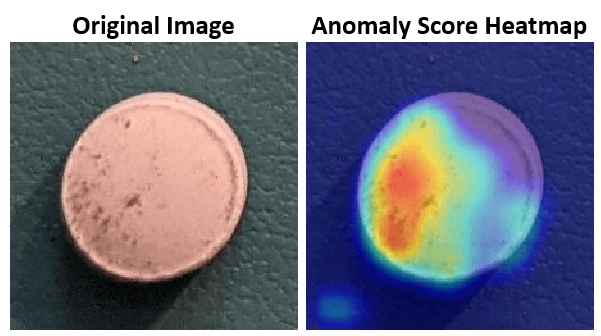This example uses the pillQC data set. The data set contains images from three classes: `normal` images without defects, `chip` images with chip defects in the pills, and `dirt` images with dirt contamination. The data set provides 149 `normal` images, 43 `chip` images, and 138 `dirt` images. The size of the data set is 3.57 MB.

Set `dataDir` as the desired location of the data set. Download the data set using the `downloadPillQCData` helper function. This function is attached to the example as a supporting file. The function downloads a ZIP file and extracts the data into the subdirectories `chip`, `dirt`, and `normal`.

```dataDir = fullfile(tempdir,"PillDefects"); downloadPillQCData(dataDir)```

This image shows an example image from each class. A normal pill with no defects is on the left, a pill contaminated with dirt is in the middle, and a pill with a chip defect is on the right. While the images in this data set contain instances of shadows, focus blurring, and background color variation, the approach used in this example is robust to these image acquisition artifacts.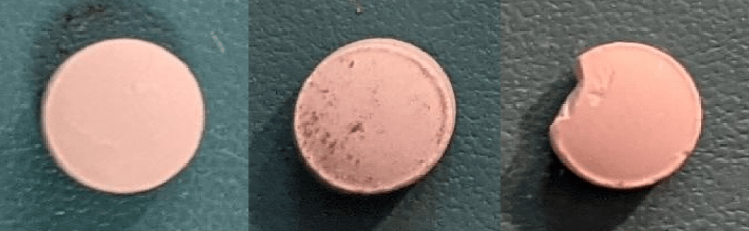Create an `imageDatastore` that reads and manages the image data. Label each image as `chip`, `dirt`, or `normal` according to the name of its directory.

```imageDir = fullfile(dataDir,"pillQC-main","images"); imds = imageDatastore(imageDir,IncludeSubfolders=true,LabelSource="foldernames");```

#### Partition Data into Training, Calibration, and Test Sets

To simulate a more typical semisupervised workflow, create a training set of 70 images from the `normal` class. Include two anomalous training images from each of the `chip` and `dirt` classes to get better classification results. Allocate 30 normal images and 15 images from each anomalous class to a calibration set. This example uses a calibration set to pick a threshold for the classifier. The classifier labels images with anomaly scores above the threshold as anomalous. Using separate calibration and test sets avoids information leaking from the test set into the design of the classifier. Assign the remaining images to the test set.

```numTrainNormal = 70; numTrainAnomaly = 2; numCalNormal = 30; numCalAnomaly = 15; [imdsNormalTrain,imdsNormalCal,imdsNormalTest] = splitEachLabel(imds,numTrainNormal,numCalNormal,"randomized",Include="normal"); [imdsAnomalyTrain,imdsAnomalyCal,imdsAnomalyTest] = splitEachLabel(imds,numTrainAnomaly,numCalAnomaly,"randomized",Include=["chip","dirt"]); imdsTrain = imageDatastore(vertcat(imdsNormalTrain.Files,imdsAnomalyTrain.Files),LabelSource="foldernames",IncludeSubfolders=true); imdsCal = imageDatastore(vertcat(imdsNormalCal.Files,imdsAnomalyCal.Files),LabelSource="foldernames",IncludeSubfolders=true); imdsTest = imageDatastore(vertcat(imdsNormalTest.Files,imdsAnomalyTest.Files),LabelSource="foldernames",IncludeSubfolders=true); trainLabels = countlabels(imdsTrain.Labels)```
```trainLabels=3×3 table Label Count Percent ______ _____ _______ chip 2 2.7027 dirt 2 2.7027 normal 70 94.595 ```

#### Augment Training Data

Augment the training data by using the `transform` function with custom preprocessing operations specified by the helper functions `augmentDataForPillAnomalyDetector` and `addConfettiNoiseForPillAnomalyDetector`. The helper functions are attached to the example as supporting files.

The `augmentDataForPillAnomalyDetector` function randomly applies 90 degree rotation and horizontal and vertical reflection to each input image. The `addConfettiNoiseForPillAnomalyDetector` function adds confetti noise to simulate local anomalies in normal images. Each normal image has a 50% likelihood of having confetti noise added to it. This augmentation step balances the training data between normal and anomalous, which helps to stabilize the loss function during training. Balancing the training data using simulated anomaly images is useful in applications where anomaly images are scarce.

```dsTrain = transform(imdsTrain,@augmentDataForPillAnomalyDetector); dsTrain = transform(dsTrain,@addConfettiNoiseForPillAnomalyDetector,IncludeInfo=true);```

Add binary labels to the calibration and test data sets by using the `transform` function with the operations specified by the `addLabelData` helper function. The helper function is defined at the end of this example, and assigns images in the `normal` class a binary label `0` and images in the `chip` or `dirt` classes a binary label `1`.

```dsCal = transform(imdsCal,@addLabelData,IncludeInfo=true); dsTest = transform(imdsTest,@addLabelData,IncludeInfo=true);```

Visualize a sample of nine augmented training images. Approximately half of the total training images have confetti noise anomalies.

```exampleData = readall(subset(dsTrain,1:9)); montage(exampleData(:,1));```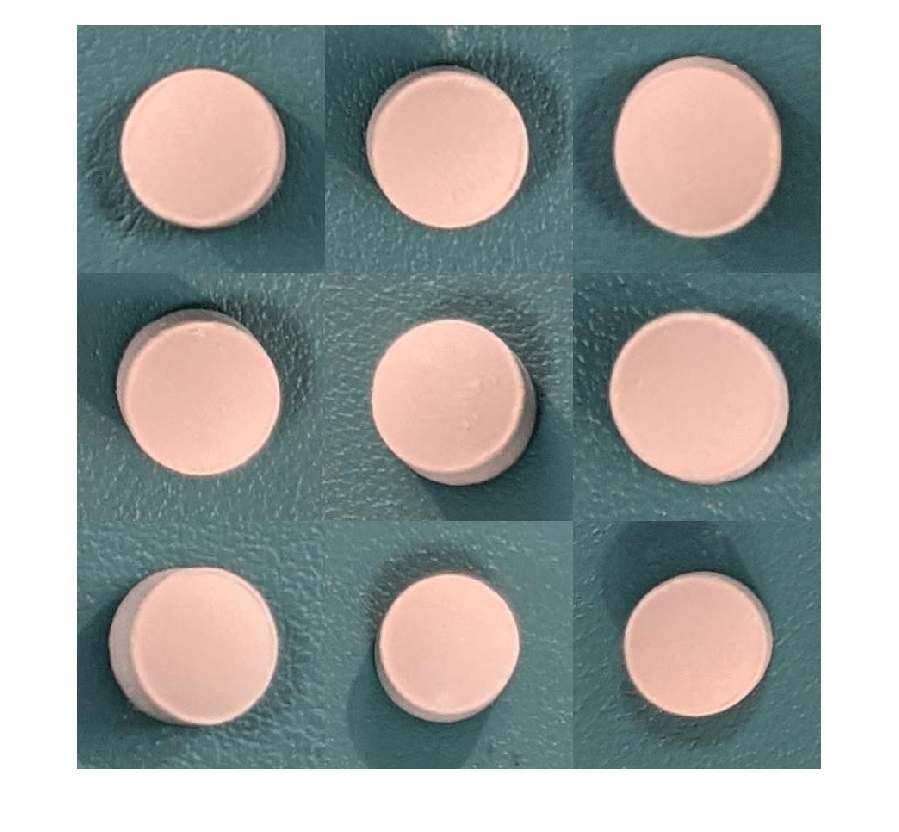### Create FCDD Model

This example uses a fully convolutional data description (FCDD) model . The basic idea of FCDD is to train a network to produce an anomaly score map that describes the probability that each region in the input image contains anomaly content.

This example uses a VGG-16 network  trained on ImageNet  as the base fully convolutional network architecture. The example freezes the majority of the model and randomly initializes and trains the final convolutional stages. This approach enables quick training with small amounts of input training data.

The `vgg16` function returns a pretrained VGG-16 network. This function requires the Deep Learning Toolbox™ Model for VGG-16 Network support package. If this support package is not installed, then the function provides a download link.

`net = vgg16;`

Replace the image input layer in the encoder with a new input layer that performs zero-center normalization using the computed mean. Set the input size of the network equal to the size of the images in the data set. Freeze the first 24 layers of the network by using the `freezeLayers` helper function. The helper function is defined at the end of this example.

```inputSize = [225 225 3]; pretrainedVGG = [imageInputLayer(inputSize,Name="input",Normalization="zerocenter") net.Layers(2:24)]; pretrainedVGG = freezeLayers(pretrainedVGG);```

Add a final convolutional stage. This stage is similar to the next convolutional stage of VGG-16 but has randomly initialized and trainable convolutional layers and with batch normalization. A 1-by-1 convolution compresses the network output into a one-channel anomaly score heatmap. The next layer is a pseudo-Huber loss function used to stabilize training with the FCDD loss and limit the output heatmap to the range [0, `inf`]  . A resize layer is used to resize the output heatmap to the same size as the input image. A global averaging pooling layer computes the scalar anomaly score as the mean of the output heatmap returned by the network. A final custom loss layer `fcddLossLayerForPillAnomalyDetector` is used to implement the loss function .

```additionalFCLayers = [ convolution2dLayer(3,512,Padding="same") batchNormalizationLayer reluLayer convolution2dLayer(3,512,Padding="same") batchNormalizationLayer reluLayer convolution2dLayer(1,1) functionLayer(@(x) sqrt(x.^2+1)-1) resize2dLayer(EnableReferenceInput=true,Method="bilinear",Name="upsampleHeatmap") globalAveragePooling2dLayer fcddLossLayer];```

Assemble the complete network.

```lgraph = layerGraph([pretrainedVGG;additionalFCLayers]); lgraph = connectLayers(lgraph,"input","upsampleHeatmap/ref");```

By default, this example downloads a pretrained version of the VGG-16 network using the helper function `downloadTrainedNetwork`. The helper function is attached to this example as a supporting file. You can use the pretrained network to run the entire example without waiting for training to complete.

To train the network, set the `doTraining` variable in the following code to `true`. Specify the number of epochs to use for training `numEpochs` by entering a value in the field. Train the model by using the `trainNetwork` function.

Train on one or more GPUs, if available. Using a GPU requires Parallel Computing Toolbox™ and a CUDA® enabled NVIDIA® GPU. For more information, see GPU Support by Release (Parallel Computing Toolbox). Training takes about 6 minutes on an NVIDIA Titan RTX™.

```doTraining =false; numEpochs=100; if doTraining options = trainingOptions("adam", ... Shuffle="every-epoch",... MaxEpochs=numEpochs,InitialLearnRate=1e-4, ... MiniBatchSize=numpartitions(dsTrain)); net = trainNetwork(dsTrain,lgraph,options); modelDateTime = string(datetime("now",Format="yyyy-MM-dd-HH-mm-ss")); save(fullfile(dataDir,"trainedPillAnomalyDetector-"+modelDateTime+".mat"),"net"); else trainedPillAnomalyDetectorNet_url = "https://ssd.mathworks.com/supportfiles/vision/data/trainedFCDDPillAnomalyDetector.zip"; downloadTrainedNetwork(trainedPillAnomalyDetectorNet_url,dataDir); net = load(fullfile(dataDir,"trainedAnomalyDetector","trainedPillFCDDNet.mat")); net = net.net; end```

### Create Classification Model

Classify an image as normal or anomalous based on whether the mean anomaly score of the image is greater than or less than a threshold value. The mean anomaly score is the average of the anomaly score heatmap. This example calculates the threshold that most accurately classifies the calibration image set.

Calculate the mean anomaly scores and known ground truth label (normal or anomaly) for each image in the calibration set.

```scores = predict(net,dsCal); labels = imdsCal.Labels ~= "normal";```

Plot a histogram of the mean anomaly scores for the normal and anomaly classes. The distributions are well separated by the model-predicted anomaly score.

```numBins = 20; [~,edges] = histcounts(scores,numBins); figure hNormal = histogram(scores(labels==0),edges); hold on hAnomaly = histogram(scores(labels==1),edges); hold off legend([hNormal,hAnomaly],"Normal","Anomaly") xlabel("Mean Anomaly Score"); ylabel("Counts");```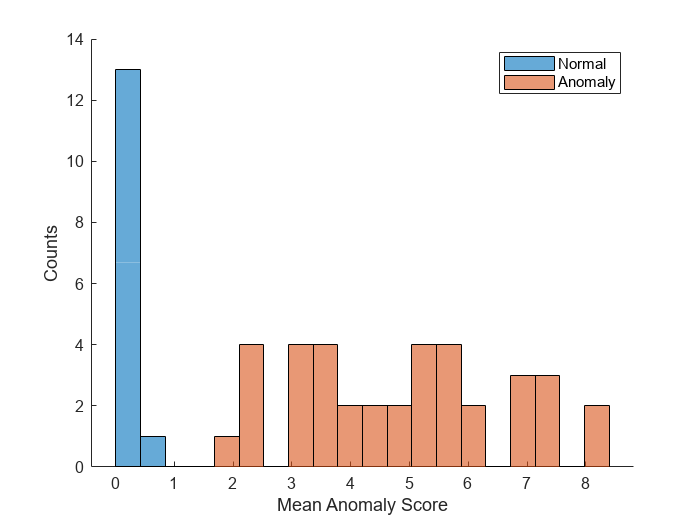Create a receiver operating characteristic (ROC) curve to calculate the anomaly threshold. Each point on the ROC curve represents the false positive rate (x-coordinate) and true positive rate (y-coordinate) when the calibration set images are classified using a different threshold value. An optimal threshold maximizes the true positive rate and minimizes the false positive rate. Using ROC curves and related metrics allows you to select a threshold based on the tradeoff between false positives and false negatives. These tradeoffs depend on the application-specific implications of misclassifying images as false positives versus false negatives.

Create the ROC curve by using the `perfcurve` (Statistics and Machine Learning Toolbox) function. The solid blue line represents the ROC curve. The red dashed line represents a random classifier corresponding to a 50% success rate. Display the area under the curve (AUC) metric for the calibration set in the title of the figure. A perfect classifier has an ROC curve with a maximum AUC of 1.

```[xroc,yroc,troc,auc] = perfcurve(labels,scores,true); figure lroc = plot(xroc,yroc); hold on lchance = plot([0 1],[0 1],"r--"); hold off xlabel("False Positive Rate") ylabel("True Positive Rate") title("ROC Curve AUC: "+auc); legend([lroc,lchance],"ROC curve","Random Chance")```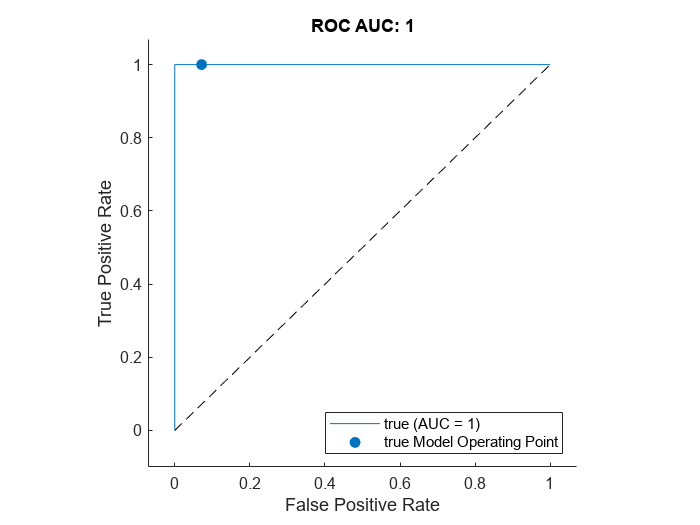This example uses the maximum Youden index metric to select the anomaly score threshold from the ROC curve. This value corresponds to the threshold that maximizes the distance between the blue model ROC curve and the red random chance ROC curve.

```[~,ind] = max(yroc-xroc); anomalyThreshold = troc(ind)```
```anomalyThreshold = single 0.3696 ```

### Evaluate Classification Model

Predict the mean anomaly score for each image in the test set. Also get the ground truth labels of each test image.

```scores = predict(net,dsTest); labels = imdsTest.Labels ~= "normal";```

Assign a class label to test images by comparing the mean anomaly scores to the threshold value.

`testSetOutputLabels = scores > anomalyThreshold;`

Calculate the confusion matrix and classification accuracy for the test set. The classification model in this example is very accurate and predicts a small percentage of false positives and false negatives.

```testSetTargetLabels = logical(labels); M = confusionmat(testSetTargetLabels,testSetOutputLabels); confusionchart(M,["Normal","Anomaly"]) acc = sum(diag(M)) / sum(M,"all"); title("Accuracy: "+acc);```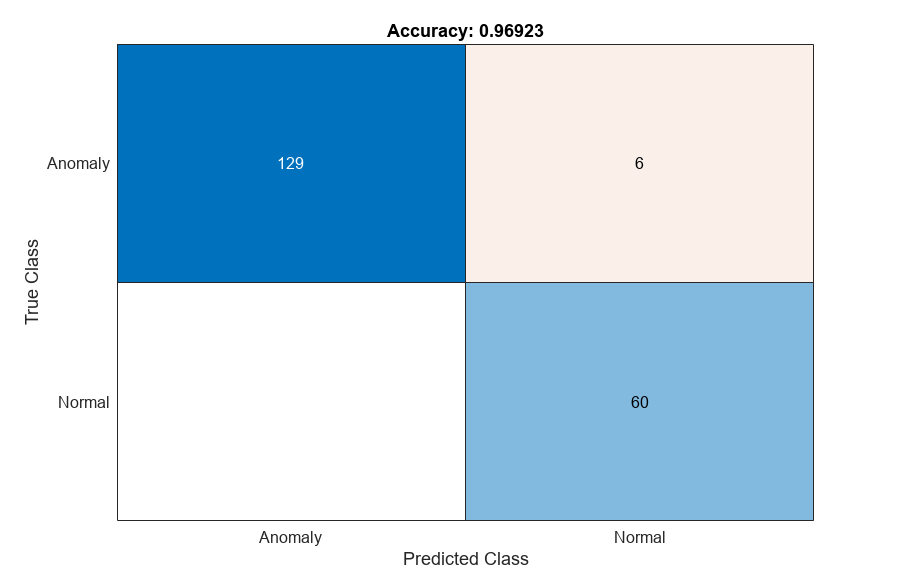### Explain Classification Decisions

You can use the anomaly heatmap predicted by the network to help explain why an image is classified as normal or anomalous. This approach is useful for identifying patterns in false negatives and false positives. You can use these patterns to identify strategies for increasing class balancing of the training data or improving the network performance.

#### View Heatmap of Anomaly

Select an image of a correctly classified anomaly. This result is a true positive classification. Display the image.

```idxTruePositive = find(testSetTargetLabels & testSetOutputLabels); dsExample = subset(dsTest,idxTruePositive); data = read(dsExample); img = data{1}; figure imshow(img)```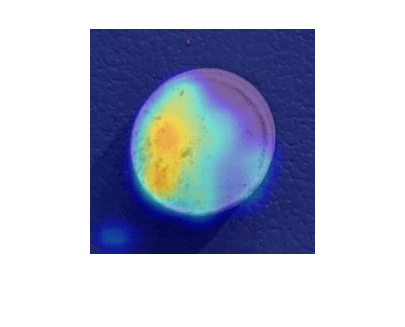Obtain a heatmap of the anomaly image by extracting the activations of the `resize2dLayer` layer `upsampleHeatmap` of the network. The resize layer returns an anomaly score heatmap the same size as the input image.

`map = activations(net,single(img),"upsampleHeatmap");`

Display an overlay of the heatmap predicted by the network over the input image by using the `heatmapOverlay` helper function. This function is defined at the end of the example. Calculate a display range that reflects the range of heatmap values observed across the entire test set. Apply the display range for all heatmaps in this example. Set the minimum value of the `displayRange` to 0. Set the maximum value to the 80th percentile value of the heatmap for the test set image with the greatest mean anomaly score. Calculate the percentile value by using the `prctile` function.

```[~,sampleIdx] = max(scores); sampleMaxScore = read(subset(dsTest,sampleIdx)); heatmapMaxScore = activations(net,sampleMaxScore{1},"upsampleHeatmap"); displayRange = [0,prctile(heatmapMaxScore,80,"all")]; imshow(heatmapOverlay(img,map,displayRange))```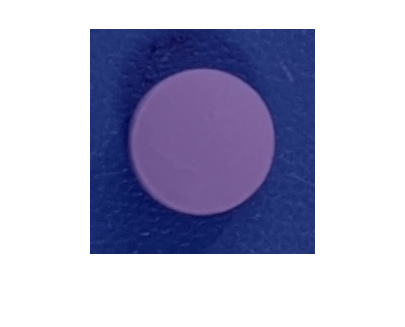To quantitatively confirm the result, display the mean anomaly score of the true positive test image as predicted by the network. The value is greater than the anomaly score threshold.

`disp("Mean heatmap anomaly score of test image: "+scores(idxTruePositive(1)));`
```Mean heatmap anomaly score of test image: 1.1949 ```

#### View Heatmap of Normal Image

Select and display an image of a correctly classified normal image. This result is a true negative classification.

```idxTrueNegative = find(~(testSetTargetLabels | testSetOutputLabels)); dsExample = subset(dsTest,idxTrueNegative); data = read(dsExample); img = data{1}; imshow(img)```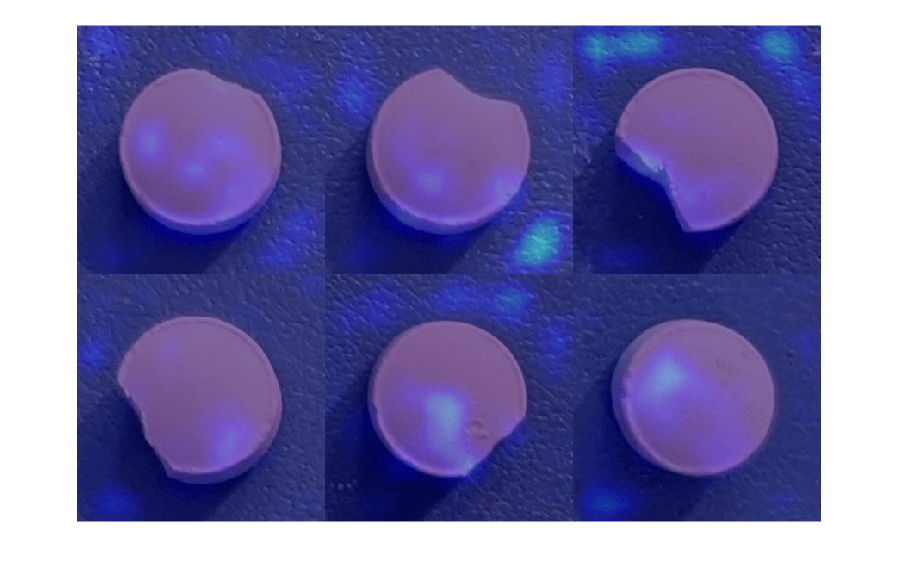Obtain a heatmap of the normal image by extracting the activations of the `resize2dLayer` layer `upsampleHeatmap` of the network. Display an overlay of the heatmap predicted by the network over the input image by using the `heatmapOverlay` helper function. This function is defined at the end of the example. Many true negative test images, such as this test image, either have no visible anomalous regions or have low anomaly scores in a localized portion of the image.

```map = activations(net,single(img),"upsampleHeatmap"); imshow(heatmapOverlay(img,map,displayRange))```Display the mean anomaly score of the true negative test image as predicted by the network. The value is less than the anomaly score threshold.

`disp("Mean heatmap anomaly score of test image: "+scores(idxTrueNegative(1)));`
```Mean heatmap anomaly score of test image: 0.12476 ```

#### View Heatmaps of False Negative Images

False negatives are images with pill defect anomalies that the network classifies as normal. Use the explanation from the network to gain insights into the misclassifications.

Find any false negative images from the test set. Obtain heatmap overlays of the false negative images by using the `transform` function. The operations of the transform are specified by an anonymous function that applies the `heatmapOverlay` helper function to the activations of the `resize2dLayer` layer `upsampleHeatmap` of the network. The `heatmapOverlay` helper function is defined at the end of the example. Display the false negative images as a montage. If there are no false negatives, the figure is empty.

```falseNegativeIdx = find(testSetTargetLabels & ~testSetOutputLabels); if ~isempty(falseNegativeIdx) fnExamples = subset(dsTest,falseNegativeIdx); fnExamplesWithHeatmapOverlays = transform(fnExamples,@(x) {heatmapOverlay(x{1},activations(net,x{1},"upsampleHeatmap"),displayRange)}); fnExamples = readall(fnExamples); fnExamples = fnExamples(:,1); fnExamplesWithHeatmapOverlays = readall(fnExamplesWithHeatmapOverlays); else [fnExamples,fnExamplesWithHeatmapOverlays] = deal([]); end montage(fnExamples)```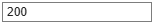Display the heatmap overlays as a montage. The network predicts visible anomaly scores around chip defects and specks of dirt, as expected.

`montage(fnExamplesWithHeatmapOverlays)`Display the mean anomaly scores of the false negative test images as predicted by the network. The mean scores are less than the anomaly score threshold, resulting in misclassifications.

`disp("Mean heatmap anomaly scores:"); scores(falseNegativeIdx)`
```Mean heatmap anomaly scores: ```
```ans = 2×1 single column vector 0.2603 0.3277 ```

#### View Heatmaps of False Positive Images

False positives are images without pill defect anomalies that the network classifies as anomalous. Use the explanation from the network to gain insights into the misclassifications.

Find any false positive images from the test set. Obtain heatmap overlays of the false positive images by using the `transform` function. The operations of the transform are specified by an anonymous function that applies the `heatmapOverlay` helper function to the activations of the `resize2dLayer` layer `upsampleHeatmap` of the network. The `heatmapOverlay` helper function is defined at the end of the example. Display the false positive images as a montage. If there are no false positives, the figure is empty.

```falsePositiveIdx = find(~testSetTargetLabels & testSetOutputLabels); if ~isempty(falsePositiveIdx) fpExamples = subset(dsTest,falsePositiveIdx); fpExamplesWithHeatmapOverlays = transform(fpExamples,@(x) {heatmapOverlay(x{1},activations(net,x{1},"upsampleHeatmap"),displayRange)}); fpExamples = readall(fpExamples); fpExamples = fpExamples(:,1); fpExamplesWithHeatmapOverlays = readall(fpExamplesWithHeatmapOverlays); else [fpExamples,fpExamplesWithHeatmapOverlays] = deal([]); end montage(fpExamples)```Display the heatmap overlays as a montage. The false positive images show regions that the network labeled as anomalous. You can use this explanation of the network behavior to gain insights into the classification problem. For example, if anomalous scores are localized to the image background, you can explore suppressing the background during preprocessing.

`montage(fpExamplesWithHeatmapOverlays)`Display the mean anomaly scores of the false positive test images as predicted by the network. The mean scores are greater than the anomaly score threshold, resulting in misclassifications.

`disp("Mean heatmap anomaly scores:"); scores(falsePositiveIdx)`
```Mean heatmap anomaly scores: ```
```ans = single 0.4467 ```

### Supporting Functions

The `freezeLayers` helper function freezes the network layers specified by the layer array `layers`.

```function layers = freezeLayers(layers) for idx = 1:length(layers) if isprop(layers(idx),"Weights") layers(idx) = setLearnRateFactor(layers(idx),Weights=0); layers(idx) = setLearnRateFactor(layers(idx),Bias=0); end end end```

The `heatmapOverlay` helper function overlays a colored heatmap `hmap` with a display range specified by `displayRange` on the image `img`.

```function out = heatmapOverlay(img,hmap,displayRange) % Normalize to the range [0, 1] img = mat2gray(img); hmap = rescale(hmap,InputMin=displayRange(1),InputMax=displayRange(2)); % Convert heatmap to an RGB image using a colormap map = jet(256); hmapRGB = ind2rgb(gray2ind(hmap,size(map,1)),map); % Blend results hmapWeight = hmap; imgWeight = 1-hmapWeight; out = im2uint8(imgWeight.*img + hmapWeight.*hmapRGB); end```

The `addLabelData` helper function creates a one-hot encoded representation of label information in `data`.

```function [data,info] = addLabelData(data,info) if info.Label == categorical("normal") onehotencoding = 0; else onehotencoding = 1; end data = {data,onehotencoding}; end```

### References

 Liznerski, Philipp, Lukas Ruff, Robert A. Vandermeulen, Billy Joe Franks, Marius Kloft, and Klaus-Robert Müller. "Explainable Deep One-Class Classification." Preprint, submitted March 18, 2021. https://arxiv.org/abs/2007.01760.

 Ruff, Lukas, Robert A. Vandermeulen, Billy Joe Franks, Klaus-Robert Müller, and Marius Kloft. "Rethinking Assumptions in Deep Anomaly Detection." Preprint, submitted May 30, 2020. https://arxiv.org/abs/2006.00339.

 Simonyan, Karen, and Andrew Zisserman. "Very Deep Convolutional Networks for Large-Scale Image Recognition." Preprint, submitted April 10, 2015. https://arxiv.org/abs/1409.1556.

 ImageNet. https://www.image-net.org.